### traffic lights, part one

24 January 2008

I spend a lot of time in traffic. Compared to many other commuters, that statement is a total lie, but for me 25 minutes stuck in a car is about 25 minutes too many.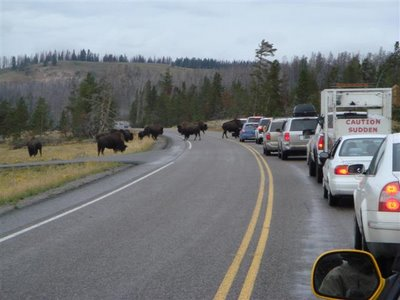And I thought the 101 was bad. (context)

I figure that if I'm stuck in traffic, I might as well not be paying for it. Recently, I upgraded to a hybrid to enhance my MPG (tax credit + gas prices == economic win), but still, I know that behaviorally there are ways to eke more distance out of gas. An easy way to save on gas (even if nominally) is to accelerate as little as possible; seeing as most of my acceleration is out from red lights, this raises the question of how do you maximize your speed through a red light, thereby reducing the amount of acceleration required to attain your desired speed?

This is an economic conundrum if ever one there was, so it makes sense to tackle it with a good measure of game theory. To make the analysis simpler, we'll start with assuming that we know when the light is going to change, and that there are no other cars in the way; in part two, when it happens, we'll look at what happens when knowledge of light-changing times follows a known probability distribution (a much more accurate model). If there is a part three, it'll examine what happens when other cars are already waiting at the light. For now, let's also assume that we're moving fast enough to run the red light in absence of acceleration (deceleration).

To start, let's issue a few definitions. Letbe the time elapsed since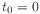; let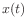be the distance to the the stopping point at time; let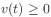and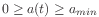be velocity and acceleration at time, respectively (naturally,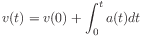; let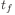be the known time at which the light is going to change. Our problem is then stated as follows: what function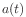maximizes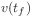subject to? That is, what acceleration function maximizes final velocity, subject to not running the light?

THEOREM: The optimal acceleration function slows as rapidly as possible to a constant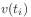, such that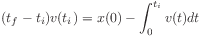. In other words, the optimal strategy is to immediately apply maxiumum braking pressure until you reach the speed which will perfectly cover the distance to the light at the time the light changes. To show this, we'll need a few tools on our belt.

LEMMA: Any optimal acceleration function must be such that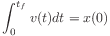. Here we declare that any velocity-maximizing acceleration function has the car crossing the line (covering distance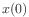) at the moment the light changes (). Suppose we have an acceleration function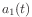— deriving velocity function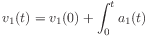— such that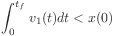. Let the derived position function be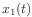.

By our assumption that we are initially moving fast enough to run the red light, we know there is some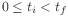such that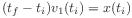, or that at some point we are moving exactly the right speed to cross the line as the light changes. We also know that there is somesuch that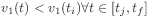; otherwise,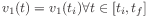, and thus we find that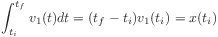, where we cross the line as the light changes, a contradiction.

Let's use our given acceleration functionto derive a new acceleration function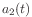:Then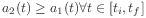(given our constraint that acceleration is negative), and more importantly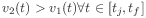. Knowing that acceleration functionhas been constructed so that we cross the line at exactly the time the light changes, and specifically that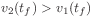, we see that from any acceleration functionsuch thatwe may derive a new acceleration functionsuch that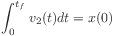and, proving our lemma.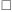Back to the theorem at hand...

Suppose we have an acceleration functionsuch that(we'll call this criterion lemma-possibly optimal, in recognition of the above lemma), and an acceleration functionwhere for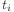such that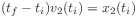Thus. Furthermore, we may see that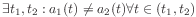; that is,anddiffer over some open interval.

By definition of, this means that for some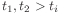we have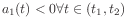. If not, then we know that for some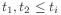we have(otherwise,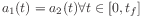, a contradiction). Then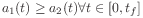and so, with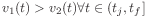for some. Then we know that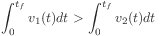, or that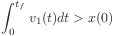, a contradiction.

Finding a suitable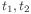as above, we see that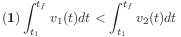; separating the lemma-possibly optimal definition integral, this implies that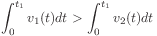. Knowing that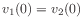and that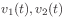are nonincreasing (by definition of the acceleration functions), we see that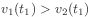. By definition of, we can use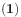to see that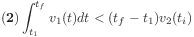. Suppose that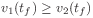, then since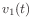is nonincreasing, we know that, a contradiction of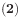.

Thus we see that for any lemma-possibly optimal acceleration function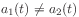,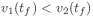. Combining this with our lemma, we have proved the theorem.### conclusion

To maximize your speed through an unblocked traffic light for which you know the change time without touching the gas pedal, slow down as quickly as possible and hold the constant intercept speed once you reach it.

Way too much math to show that little fact. Sometime soon, I'll work on deriving a similar proof for unknown change times following a probability distribution. Then we'll be cooking with gas.

All includedgraphics are generated at LaTeX to png .